output.to from Sideway

Draft for Information Only

# Content

```  Acoustic Plane Wave   Complex Exponential Representation   Plane Wave Properties   Medium Acoustic Impedance   Plane Wave Superimposition```

## Acoustic Plane Wave

For a 1D acoustic plane wave in x direction,:where

p is a function of x and t.
c is the speed of propagation.

The general solution of the equation is an arbitrary wave propagation along positive x direction and negative x direction.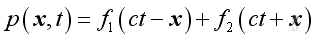For ct-x, the propagation of pressure fluctuation should be repeated after the period ct-x, therefore after time t, the wave should propagate a distance x in positive direction outwardly.

But for ct+x, the propagation of pressure fluctuation should be repeated after the period ct+x, therefore after time t, the wave should propagate a distance x in negative direction inwardly.

Since p is a function of x and t, it is characteristic by a double periodicity.

In time domain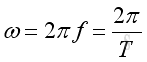where

ω is angular velocity
ƒ is frequency
ωT is period

And in physical domain: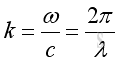where

k is wave number
c is speed of wave propagation λ is wave length

Consider a solution of the form: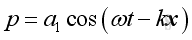where

ω is the angular frequency
k=ω/c
a1 is the magnitude constant, therefore: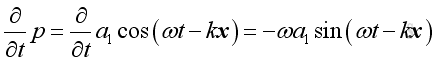,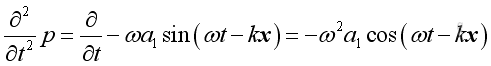and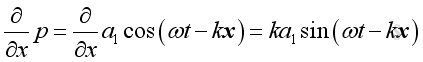,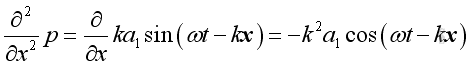Substituting into the 1D wave equation and the form of solution is confirmed.
And a solution of the same form in negative x direction is: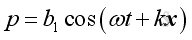Therefore the general harmonic wave solution of wave equation is: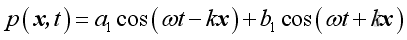Similarly, consider a solution of the form: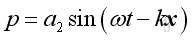where

ω is the angular frequency
k=ω/c,
a2 is the magnitude constant,
therefore: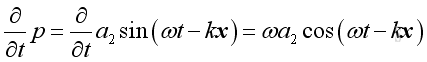,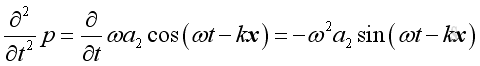and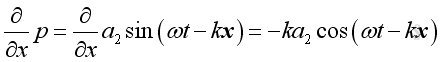,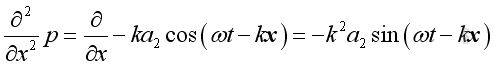Substituting into the 1D wave equation and the form of solution is confirmed also.

Since the 1D wave equation is linearized by making the differential coefficient is of first order only, the sum of two solution forms is also a solution of the wave equation. The differences between two solutions are the phase angle and the magnitude.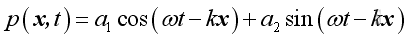The general expression of a harmonic function is: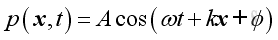and it can be decomposed into sinusoidal and cosinusoidal components in quadrature of 90 degrees out of phase: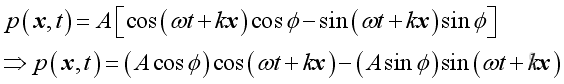These two components can be individual harmonic function with arbitrary phase angle φ as in the first two solutions. Or they can be linked solution in a linear system in which the phase angle is not an arbitrary and is related as in the above solution:

## Complex Exponential Representation

Since in complex system,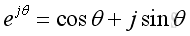The solution of wave equation can be expressed in a complex exponential form,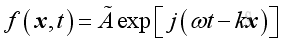where

Ã is Complex function of the form a+jb
exp[j(ωt-kx)] is ej(ωt-kx)
j is √(-1)

Since it is a complex function, the real acoustic pressure function can be represented by the real part of the complex expression.
Therefore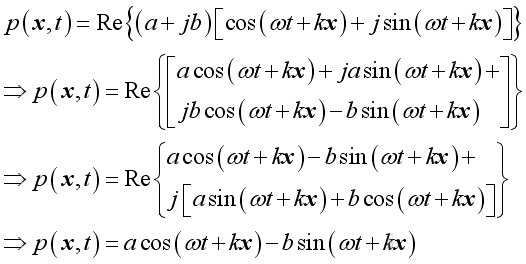By comparing with the wave equation solution, imply: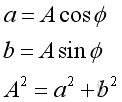Therefore, the general complex harmonic wave solution of 1D wave equation is :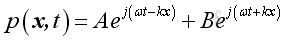## Plane Wave Properties

Consider a plane wave propagates along positive x direction: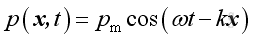or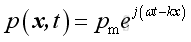From the linearized conservation of momentum, the relationship between acoustic pressure and acoustic velocity is: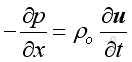Therefore: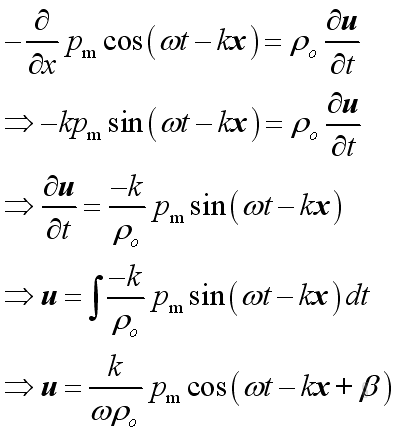The differences in magnitude between acoustic pressure and acoustic velocity are the relative magnitude and the relative phase angle.
Wave propagation is a kind of forced vibration, from the linearized conservation of momentum,  Then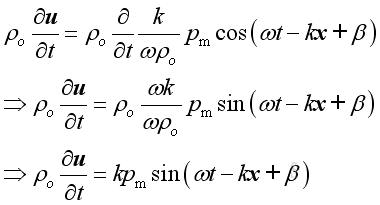or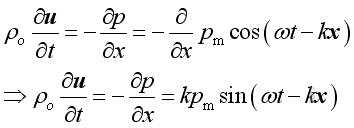Imply :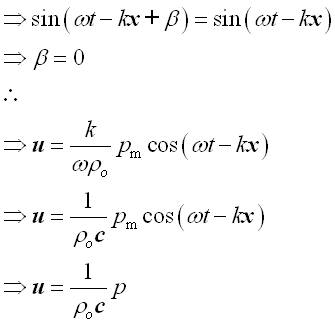Therefore the relationship between acoustic pressure and acoustic velocity is: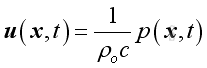In general: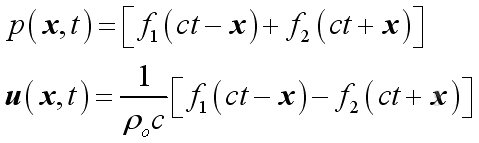## Medium Acoustic Impedance

The acoustic impedance of a medium is defined as the ratio of acoustic pressure to the acoustic velocity, Then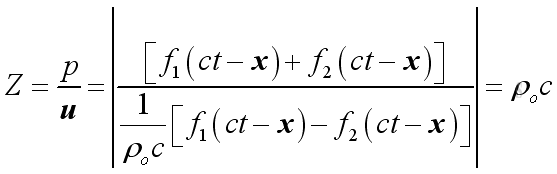For air, z=400

## Plane Wave Superimposition

Consider two harmonic plane waves propagate of the same frequency along positive x direction and negative x direction respectively. The pressure fluctuation of two harmonic plane waves i.e. A along positive x direction and B along negative x direction are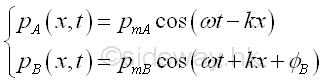If the two harmonic plane waves are in phase at x=0, then the phase angle at x is equal to zero also. Therefore the pressure fluctuation of two harmonic plane waves at x are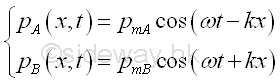The total sound pressure at x is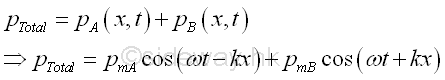According to the linearized equation of motion,Imply,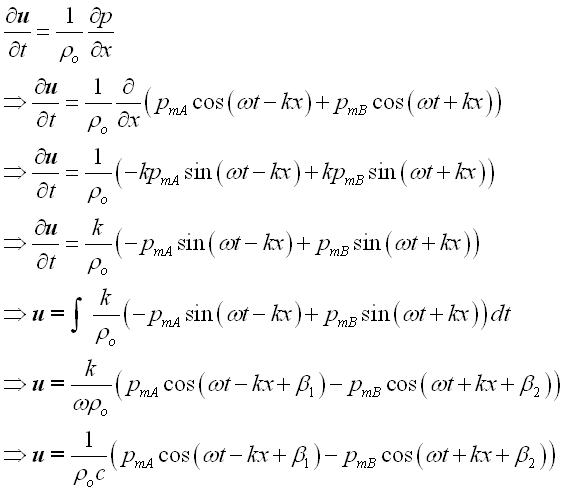From the linearized equation of motion, imply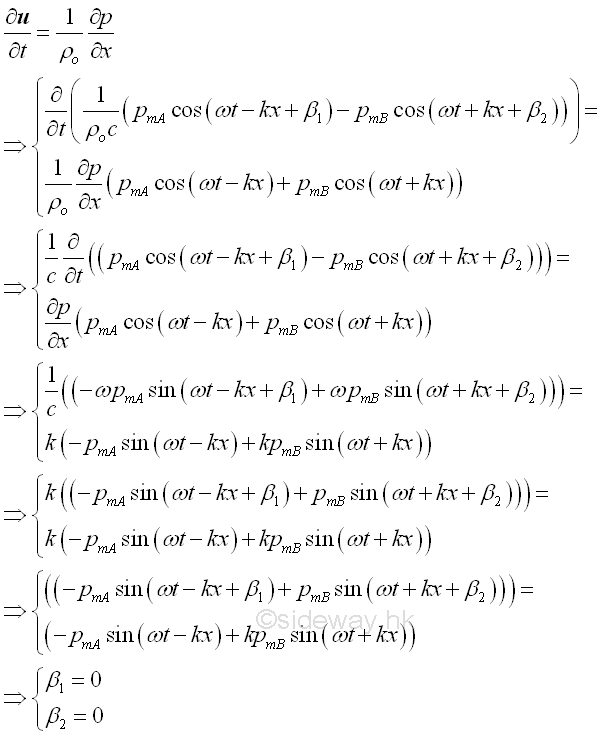Therefore, the vector sum of the particle velocity at x is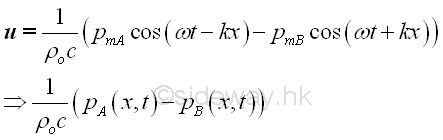©sidewayReferences

1. Michael P. Norton; Denis G. Karczub,, 2003, Fundamentals of Noise and Vibration Analysis for Engieer, Cambridge, United Kingdom
2. G. Porges, 1977, Applied Acoustics, Edward Arnold Limited, Britain
3. Douglas D. Reynolds, 1981, Engineering Principles of Acoustics:; Noise and Vibration Control, Allyn and Bacon, USA
4. Conrad J. Hemond, 1983, Engineering Acoustics & Noise Control, Prentice-Hall, USA
5. F. Fahy, 2001, Foundations of Engineering Acoustics, Academic Press, UK
6. D.A. Biew; C.H. Hansen, 1996, Engineering Noise Control: Theory and Practice, E & FN Spon, New YorkID: 100900021 Last Updated: 7/30/2012 Revision: 2 Ref:Home (5)

Business

Management

HBR (3)

Information

Recreation

Hobbies (7)

Culture

Chinese (1097)

English (336)

Reference (66)

Computer

Hardware (149)

Software

Application (187)

Digitization (24)

Numeric (19)

Programming

Web (644)CSS (SC)

ASP.NET (SC)

HTML

Knowledge Base

Common Color (SC)

Html 401 Special (SC)

OS (389)MS Windows

Windows10 (SC)

.NET Framework (SC)

DeskTop (7)

Knowledge

Mathematics

Formulas (8)

Number Theory (206)

Algebra (20)

Trigonometry (18)

Geometry (18)

Calculus (67)

Complex Analysis (21)

Engineering

Tables (8)

Mechanical

Mechanics (1)

Rigid Bodies

Statics (92)

Dynamics (37)

Fluid (5)

Control

Acoustics (19)

Biology (1)

Geography (1)

Latest Updated Links

Copyright © 2000-2019 Sideway . All rights reserved Disclaimers last modified on 10 Feb 2019